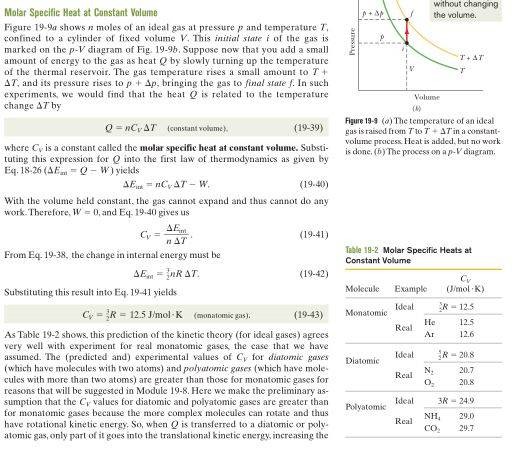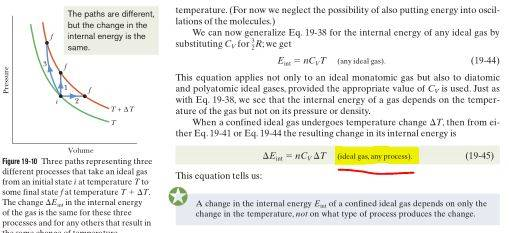# Internal Energy of an Ideal gas related to Molar specific heat

## Homework Statement

Please look at the below images which is the derivation of the relation between the internal energy of an ideal gas and the molar specific heat at constant volume. (Snaps taken from Fundamentals of Physics
Textbook by David Halliday, Jearl Walker, and Robert Resnick)As the above highlighted part suggests, the equation derived is valid for all processes (given that the gas is an ideal gas), however, the assumptions in the initial phase (or eq.19-39) of the derivation suggest that the process we are studying is at a constant volume and thus, the equation derived should be valid for processes involving constant volume.
Please throw light as to where I am wrong.

## Answers and Replies

kuruman
Science Advisor
Homework Helper
Gold Member
What is equation 19-38? I don't have the book. Anyway, the change in internal energy depends only on the initial and final temperature not on how you get from one to the other. That's because the internal energy depends on T only.

•SciencyBoi
Chestermiller
Mentor
For materials in general, the internal energy is a function of both temperature and specific volume. However, for an ideal gas, the internal energy depends only on temperature.

There is a bit of slight of hand in the image you provided. In freshman physics we learned that the heat Q is equal to the mass times the specific heat capacity times the temperature change. Now, in thermodynamics, we learn that Q depends on path, and so the relationship no longer applies. Instead, we are taught an expanded definition involving either constant volume or constant pressure. The thermodynamic definitions of the heat capacities are as follows: $$\left(\frac{\partial U}{\partial T}\right)_v=mC_v$$and$$\left(\frac{\partial H}{\partial T}\right)_v=mC_p$$.These definitions are consistent with the freshman physics versions if the system is either at constant volume or at constant pressure. The subscripts v and p refer to how the heat capacities can be measured experimentally (by determining the heat Q either at constant volume or at constant pressure), rather than by how these heat capacities are applied in practice to solve problems in which the volume or pressure may be varying.

•SciencyBoi